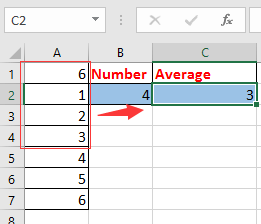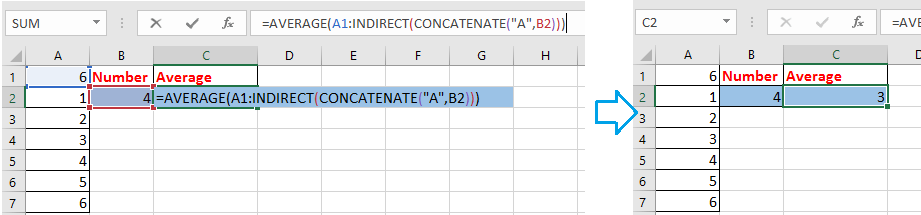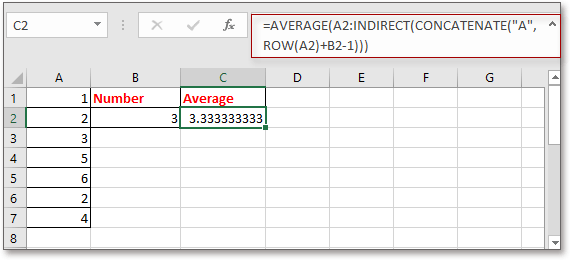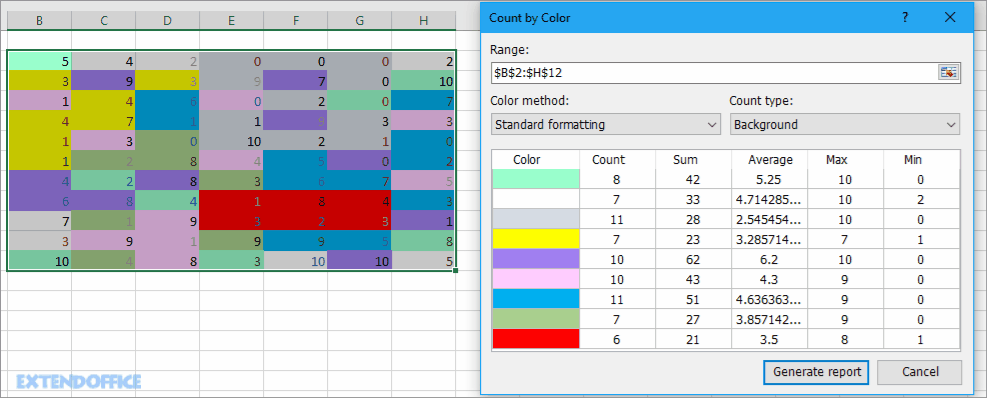## 如何在Excel中基於另一個單元格值定義範圍？####根據單元格值定義範圍1.在公式中，A1是要計算的列中的第一個單元格，A是要計算的列，B2是要基於其計算的單元格。 您可以根據需要更改這些引用。

2.如果要進行匯總，則可以使用此公式 = SUM（A1：INDIRECT（CONCATENATE（“ A”，B2）））.

3.如果要定義的第一個數據不在Excel的第一行中，例如在單元格A2中，則可以使用以下公式： =平均（A2：INDIRECT（CONCATENATE（“ A”，ROW（A2）+ B2-1）））.#### 在Excel中按背景或格式顏色快速計數/求和單元格Kutools for Excel：超過300個方便的Excel插件，免費試用，沒有限制 30 天。

### 最佳辦公生產力工具

 熱門特色: 尋找、突出顯示或識別重複項   |  刪除空白行   |  合併列或儲存格而不遺失數據   |   沒有公式的回合 ... 超級查詢: 多條件VLookup  |   多值VLookup  |   跨多個工作表的 VLookup   |   模糊查詢 .... 高級下拉列表: 快速建立下拉列表   |  依賴下拉列表   |  多選下拉列表 .... 欄目經理: 新增特定數量的列  |  移動列  |  切換隱藏列的可見性狀態  |  比較範圍和列 ... 特色功能: 網格焦點   |  設計圖   |   大方程式酒吧   |  工作簿和工作表管理器   |  資源庫 （自動文字）   |  日期選擇器   |  合併工作表   |  加密/解密單元格   |  按清單發送電子郵件   |  超級濾鏡   |   特殊過濾器 （過濾粗體/斜體/刪除線...）... 前 15 個工具集:  12 文本 工具 (添加文本, 刪除字符，...）   |   50+ 圖表 類型 (甘特圖，...）   |   40+ 實用 公式 (根據生日計算年齡，...）   |   19 插入 工具 (插入二維碼, 從路徑插入圖片，...）   |   12 轉化 工具 (數字到單詞, 貨幣兌換，...）   |   7 合併與拆分 工具 (高級合併行, 分裂細胞，...）   |   ... 和更多#### Office Tab 為 Office 帶來選項卡式界面，讓您的工作更輕鬆

• 在Word，Excel，PowerPoint中啟用選項卡式編輯和閱讀，發布者，Access，Visio和Project。
• 在同一窗口的新選項卡中而不是在新窗口中打開並創建多個文檔。
• 將您的工作效率提高 50%，每天為您減少數百次鼠標點擊！No ratings yet. Be the first to rate!
This comment was minimized by the moderator on the site
But If I want to sum a column range(say l7:l7800) out of the range d7:ct9000 based on the condition in column a(a7:a9000) and column b(b7:b9000). How will I do it. Please advise
This comment was minimized by the moderator on the site
Is there a way to create a dynamic ranged view, that will isolate all orders from a specific client within a list of clients? I have looked at multiple videos and none actually do what I need it to do. Is there a way to do this or would I need to pull the needed info into a controlled table and use the information from that table?
This comment was minimized by the moderator on the site
Hi, CareTaker. I do not get your question clearly. Could you describe your question or upload a file to show the quetion in details? But I recomend a utility called Data Association for you, maybe can help you, go to this web for details: https://www.extendoffice.com/product/kutools-for-excel/excel-click-cell-to-filter-based-on-another-workbook.html.
This comment was minimized by the moderator on the site
Thank you for the response, its quite the complicated thing to explain but I did manage to come right in the end using the indexing formula with the match formula as well.   =INDEX(\$B:\$B;MATCH(\$C\$2;\$B:\$B;0);):INDEX(\$N:\$N;MATCH(\$C\$2;\$B:\$B;0)+\$C\$3-1;)   at first it was confusing but I'm good now
This comment was minimized by the moderator on the site
how do I update sum range by using helper cell...For example: if initial sum range is = C1 to M1, how to do change sum range if new data is up to AB1 using a helper cell, instead of changing the formula manually?
This comment was minimized by the moderator on the site
What if the rage is not column, but a row?
This comment was minimized by the moderator on the site
That's a bit trickier as I just found out. You need to use a combination of the CHAR function to convert a number to a letter, CONCAT to piece together a letter and number comprising a cell address, and the INDIRECT function to recognize strings as cell addresses.

In my example, I wrote the following expression:

``````=SUM(INDIRECT(CONCAT("F",ROW())):INDIRECT(CONCAT(CHAR(64+5+\$B\$31),ROW())))
``````

where:
Row() is the current row
\$B\$31 is the cell where the # of columns to count is stored
5 is the offset for the first column to be counted

if you need to go beyond column Z, you’d need a less intuitive variant on that expression using the ADDRESS function:

``=SUM(indirect(concat("F",row())):indirect(ADDRESS(row(),\$B\$31+5,4)))``

where:
4 indicates a relative reference
This comment was minimized by the moderator on the site
Hi quick question. My formula =SUMIFS(DATA!\$D\$5:\$D\$13914,DATA!\$E\$5:INDIRECT(CONCATENATE("\$E\$",C3)),"<"&"1/1/2016") has a range that is from another tab called "DATA." I keep getting #VALUE! errors for this formula. Any suggestions? thanks!
This comment was minimized by the moderator on the site
Earlier I posted: Great formula but why does it only work when referring to the first cell. In other words, if you move the whole range down and the first cell in the range is A5 instead of A1, the formula ceases to work.
After playing with it, I figured out why the formula is not working when the starting row is not row 1. Excel interprets this as the range being A1 thru A4. If you are starting with a different row than row 1, here is how to modify the formula assuming the range now starts with A5:=MAX(A5:INDIRECT(CONCATENATE("A",ROW(A5)+B2)))
This works because the concatenation sees the row reference as fixed in the first example, but it becomes relative in the second example. Hope that helps!
Jared - You can just subtract 4 instead of adding. =MAX(A5:INDIRECT(CONCATENATE("A",ROW(A5)-B2))) [Assuming you have 4 in cell B2, or you could just leave the formula as is and put -4 in cell B2.]
This comment was minimized by the moderator on the site
Hi，cathy, thanks for your supplement, I have test your formula, I think the correct formula may be =MAX(A5:INDIRECT(CONCATENATE("A",ROW(A5)+B2-1))). I have update the tutorual. Thanks again.
This comment was minimized by the moderator on the site
Great formula but why does it only work when referring to the first cell. In other words, if you move the whole range down and the first cell in the range is A6 instead of A1, the formula ceases to work. That seems strange.
This comment was minimized by the moderator on the site
What if I want to count upward from a cell rather than downward? In the above example, the formula starts with A1 and goes downward. Let's say I want a range of 4 cells, beginning with Cell A10 and going to A6. Thank you!
This comment was minimized by the moderator on the site
Jared - See my most recent post for how to solve this.
This comment was minimized by the moderator on the site
I'm having this upward problem also but unable to see your other post for the solution. Can you give me link of the post please? Many thanks!
This comment was minimized by the moderator on the site
=AVERAGE(INDIRECT(CONCATENATE("A",COUNT(A:A)-B1+1)):INDIRECT(CONCATENATE("A",COUNT(A:A))))
A:A is the column that you want to calcualte the average values, B1 is the cell that define the range in column A to calculate.
This comment was minimized by the moderator on the site
Thanks, but may I know why need to use "-B1+1" please?
This comment was minimized by the moderator on the site
Hi, Let me take a detailed example to explain it for you. There are 10 rows in column A, I want to get the last 2 values' average, in other words, average the values in row 9 and row 10. Now B1 contains 2, =COUNT(A:A)-B1+1 will get the result 9, COUNT(A:A) will get the result 10 (the last row of the column A). Now combine other functions to averaget the values in row 9 and row 10. Hope this can help you.
This comment was minimized by the moderator on the site
Can I use this syntax while referring to a different sheet?
This comment was minimized by the moderator on the site
If you want to sum referring to a Different Shet In CONCATENATE formula write [ SHEET NAME+COLUMN+ROW]
Formula=SUM(INDIRECT(CONCATENATE("Sheet1!","D",1)):INDIRECT(CONCATENATE("Sheet1!","D",5)))
This comment was minimized by the moderator on the site
Hi, Thank you so much for your help, but I have a small problem that is I don't have just one column but several ones (about 100 columns) is there a way to change the formula to accommodate the change in columns so O can get the sum for Columns A B C and so on. thanks for your help
This comment was minimized by the moderator on the site
Thank u for your message. If you want to sum first n values across multiple continuous columns, just change A to the last column you ues. EG, sum first 5 values across column A to column D, apply this formula =SUM(A1:INDIRECT(CONCATENATE("D",F2))).
There are no comments posted here yet